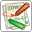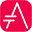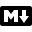#Edit in DokuwikiEdit in AsciidocEdit in MarkdownMaths

Within PlantUML, you can use AsciiMath notation:

 🎉 Copied!@startuml :$int_0^1f(x)dx$; :$x^2+y_1+z_12^34$; note right Try also $d/dxf(x)=lim_(h->0)(f(x+h)-f(x))/h$ $P(y|bb"x") or f(bb"x")+epsilon$ end note @endumlor JLaTeXMath notation:

 🎉 Copied!@startuml :\int_0^1f(x)dx; :x^2+y_1+z_{12}^{34}; note right Try also \dfrac{d}{dx}f(x)=\lim\limits_{h \to 0}\dfrac{f(x+h)-f(x)}{h} P(y|\mathbf{x}) \mbox{ or } f(\mathbf{x})+\epsilon end note @endumlHere is another example:

 🎉 Copied!@startuml Bob -> Alice : Can you solve: $ax^2+bx+c=0$ Alice --> Bob: $x = (-b+-sqrt(b^2-4ac))/(2a)$ @enduml##Edit in DokuwikiEdit in AsciidocEdit in MarkdownStandalone diagram

You can also use @startmath/@endmath to create standalone AsciiMath formula.

 🎉 Copied!@startmath f(t)=(a_0)/2 + sum_(n=1)^ooa_ncos((npit)/L)+sum_(n=1)^oo b_n\ sin((npit)/L) @endmathOr use @startlatex/@endlatex to create standalone JLaTeXMath formula.

 🎉 Copied!@startlatex \sum_{i=0}^{n-1} (a_i + b_i^2) @endlatex##Edit in DokuwikiEdit in AsciidocEdit in MarkdownHow is this working?

To draw those formulas, PlantUML uses two open source projects:

• AsciiMath that converts AsciiMath notation to LaTeX expression;
• JLatexMath that displays mathematical formulas written in LaTeX. JLaTeXMath is the best Java library to display LaTeX code.

ASCIIMathTeXImg.js is small enough to be integrated into PlantUML standard distribution.

Since JLatexMath is bigger, you have to download it separately, then unzip the 4 jar files (batik-all-1.7.jar, jlatexmath-minimal-1.0.3.jar, jlm_cyrillic.jar and jlm_greek.jar) in the same folder as PlantUML.jar.